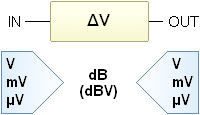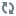# Conversion voltage gain or attenuation to decibel, dB (dBV).It converts a voltage difference in the corrisponding dB value of gain or attenuation.

Data input

Initial voltage value . . . . . IN

V

Final voltage value . . . OUT

VResults Voltage ratio Gain dB

Notes.
You can use any measure unit of power but it must be the same for the two inserted numbers.

Some reference levels :

• 0 dBµV = 1 µV su 75 ohm
• 0 dBmV = 1 mV su 75 ohm
• 0 dBm, dBmW = 1 mW
• 0 dBW = 1 W

Others :

• 0 dBj = 1 mV
• 0 dBk = 1 kW
• 0 dBs = 1 mW
• 0 VU = 1 mW

Instructions
Want to help us improve ?
Notices